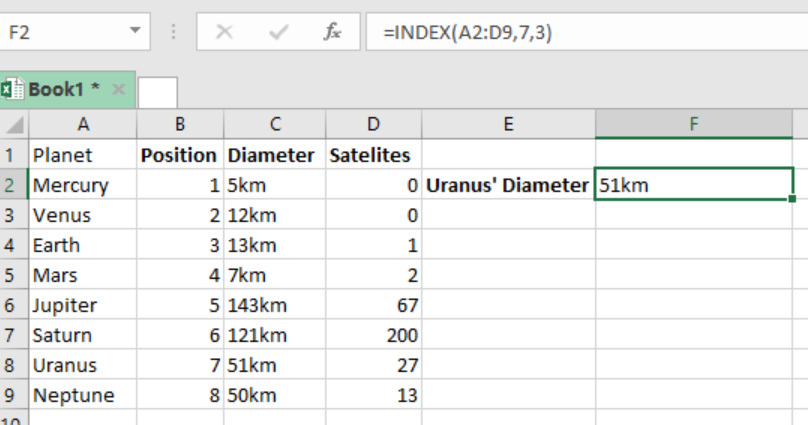Get instant live expert help with Excel or Google Sheets“My Excelchat expert helped me in less than 20 minutes, saving me what would have been 5 hours of work!”

#### Post your problem and you’ll get Expert help in seconds.

Your message must be at least 40 characters
Our professional Expert are available now. Your privacy is guaranteed.

# Excel INDEX Function

We can use the Excel INDEX function to get the value at a particular position in a given range or array.  We can also use this function to retrieve individual values, entire rows or columns. This post provides a guide on how to use the Excel INDEX function to get the value at a particular position in an array or range in excel spreadsheet.Figure 1: Using Excel INDEX function

## General syntax of the formula

`=INDEX (array, row_num, [col_num], [area_num])`

Where;

•         Array- range of cells
•         Row_num- row position in the array
•         Col_num- column position in the array, it’s optional
•         Area_num- range in the reference to be used, optional as well.

## Understanding the Excel INDEX function

The Excel INDEX function is fundamental when we want to get a value in a list or table, based on the position of that value. This function can as well help us get the individual values, rows or columns in a table.

Usually, the INDEX function is used together with the MATCH function. When used with the MATCH function, the MATCH function will only help to locate and provide the location to the INDEX function.

There are two formats of the INDEX function;

• The array form
• Reference form

## Array form

In the array form, the syntax looks like the one below;

`=INDEX (array, row_num, [col_num])`

Here, you should note the following;

• The INDEX function will return the value in the cell that interjects the row_num and col_num if we have both the row and column numbers.
• When row_num is zero, the function will return an array of values for the whole row.
• The array values can only be used by entering the INDEX function as an array formula in horizontal range. It can also be fed into another function.
• When the col_num is zero, INDEX function will return an array of values for the whole column.
• The array values can only be used by entering the INDEX function as an array formula in a vertical range. It can also be fed into another function.

## Reference form

Here, the syntax will look as below;

`=INDEX (reference row_num, [col_num], [area_num])`

• In this format, the INDEX function will return the reference of the cell where the row_num and col_num intersects.
• When reference is supplied as a multiple ranges, area_num will indicate range to be used.
• Area_sum is a number

Most of the time, the problem you will need to solve will be more complex than a simple application of a formula or function. If you want to save hours of research and frustration, try our live Excelchat service! Our Excel Experts are available 24/7 to answer any Excel question you may have. We guarantee a connection within 30 seconds and a customized solution within 20 minutes.

### Did this post not answer your question? Get a solution from connecting with the expert.Another blog reader asked this question today on Excelchat:## Subscribe to Excelchat.coAnother blog reader asked this question today on Excelchat: